## Content description

Choose appropriate units of measurement for length, area, volume, capacity and mass (ACMMG108)

Calculate the perimeter and area of rectangles using familiar metric units (ACMMG109)

Source: Australian Curriculum, Assessment and Reporting Authority (ACARA)

### What is perimeter?

The word 'perimeter' comes from two Greek words; peri, meaning 'around', and metron, meaning 'measure'. So perimeter means the measure or distance around something. It is the length around the edge.

The perimeter of a straight-sided two-dimensional shape is the sum of the lengths of its sides.

 So we can calculate the perimeter of this shape: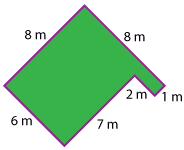Detailed description Perimeter = 8 m + 8 m + 1 m + 2 m + 7 m + 6 m= 32 m We can calculate the perimeter of this square: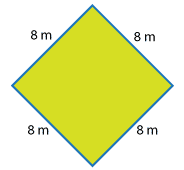P = 8 m + 8 m + 8 m + 8 m= 32 m We can calculate the perimeter of this rectangle: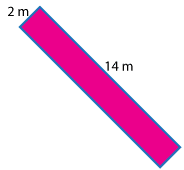P = 2 m + 14 m + 2 m + 14 m= 32 m We can calculate the perimeter of this octagon: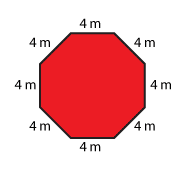P = 4 m + 4 m + 4 m + 4 m + 4 m + 4 m + 4 m + 4 m= 32 m We can calculate the perimeter of this triangle: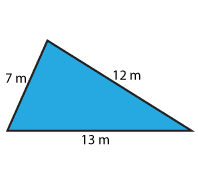P = 7 m + 12 m + 13 m= 32 m

You might have noticed that the different shapes have the same perimeter.